### Graphs Class 8th Mathematics Term 2 Tamilnadu Board Solution

##### Question 1.Draw a linear graph for the following data.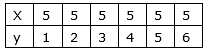Answer:When x = 5, y = 1 consider it as point A with co-ordinates (5, 1)When x = 5, y = 2 consider it as point B with co-ordinates (5, 2)When x = 5, y = 3 consider it as point C with co-ordinates (5, 3)When x = 5, y = 4 consider it as point D with co-ordinates (5,4)When x = 5, y = 5 consider it as point E with co-ordinates (5, 5)When x = 5, y = 6 consider it as point F with co-ordinates (5, 6)Plot the points A, B, C, D, E and F and join them to get the required linear graph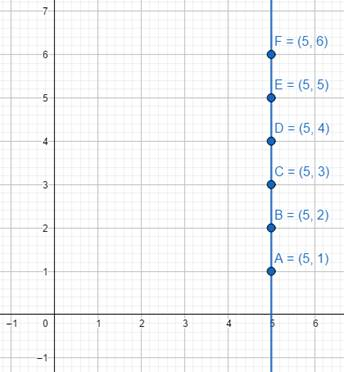Question 2.Draw a linear graph for the following data.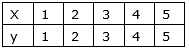Answer:When x = 1, y = 1 consider it as point A with co-ordinates (1, 1)When x = 2, y = 2 consider it as point B with co-ordinates (2, 2)When x = 3, y = 3 consider it as point C with co-ordinates (3, 3)When x = 4, y = 4 consider it as point D with co-ordinates (4,4)When x = 5, y = 5 consider it as point E with co-ordinates (5, 5)Plot the points A, B, C, D, E and F and join them to get the required linear graph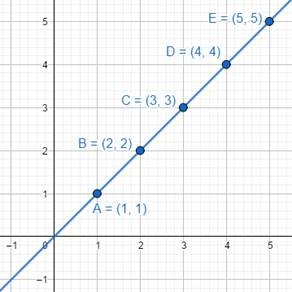Question 3.Draw the linear graph and find the missing entries.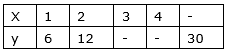Answer:Consider the missing entries as a, b and c as follows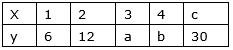As given the graph is linear i.e. it would be straight lineWhen x = 1, y = 6 consider it as point A with co-ordinates (1, 6)When x = 2, y = 12 consider it as point B with co-ordinates (2,12)Plot points A and B and draw a straight line passing through it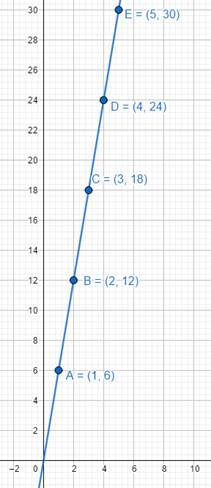When x = 3, y = a consider it as point C with co-ordinates (3, a)But from graph point C has co-ordinates (3,18)⇒ a = 18When x = 4, y = b consider it as point D with co-ordinates (4, d)But from graph point D has co-ordinates (4, 24)⇒ b = 24When x = c, y = 30 consider it as point E with co-ordinates (c, 30)But from graph point C has co-ordinates (5,30)⇒ c = 30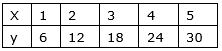Question 4.Draw the following graph of side–area relationship of a square.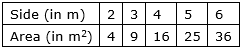Answer:Consider size as x co-ordinate and area as y co-ordinateWhen x = 2, y = 4 consider it as point A with co-ordinates (2, 4)When x = 3, y = 9 consider it as point B with co-ordinates (3, 9)When x = 4, y = 16 consider it as point C with co-ordinates (4, 16)When x = 5, y = 25 consider it as point D with co-ordinates (5,25)When x = 6, y = 36 consider it as point E with co-ordinates (6,36)Plot points A, B, C, D and E and join them to get the required graph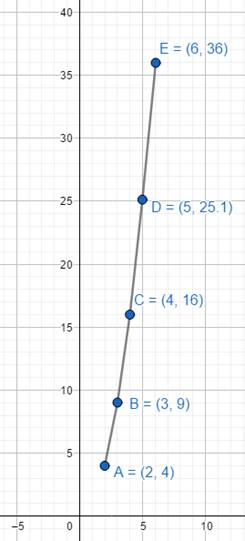Question 5.Draw the graph of y = 7x.Answer:y = 7x is a linear equation therefore its graph would be a straight linenow to draw a straight line we require atleast two pointsconsider any value of x and put it in y = 7x to get corresponding value of ylet x = 0 after putting x = 0 in y = 7x we get y = 0 consider this point as A with co-ordinates (0, 0)let x = 1 after putting x = 1 in y = 7x we get y = 7 consider this point as B with co-ordinates (1, 7)plot points A and B and draw a line passing through them, we get graph of y = 7x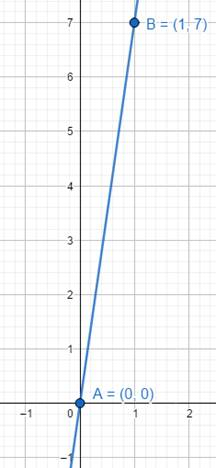Question 6.If Akbar is driving a car at a uniform speed of 40 km/hr. Draw the distance time graph. Also find the time taken by Akbar to cover a distance of 200 km.Answer:Seed is uniform i.e. 40 km/hrWhich means Akbar covers 40 km distance in 1 hourUsing speed =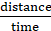So, in two hours Akbar will travel 40 × 2 = 80 km distanceLet ‘a’ be the time required to cover 200 km⇒ 40 =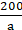⇒ a =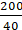⇒ a = 5 hoursRepresenting in tabular form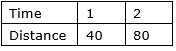Consider time on X-axis and distance on Y-axisConsider point A with co-ordinates (1, 40) and point B with co-ordinates (2, 80)Plot points A and B and draw a line passing through them is the required graphScale:On X-axis 1 cm = 1 hourOn Y-axis 1 cm = 10 km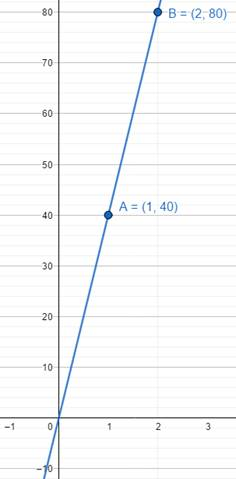Question 7.Eliza deposited ₹ 20,000 in a bank at the rate of 10% per annum. Draw a linear graph showing the time and simple interest relationship. Also, find the simple interest for 4 years.Answer:Principal amount = P = 20,000 rsRate = r = 10%Time = tSimple interest = I =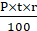⇒ I =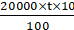⇒ I = 2000t …(i)Simple interest for 4 years implies t = 4Put t = 4 in equation (i)⇒ I = 2000 × 4⇒ I = 8000 rsConsider equation (i)Linear graph means a straight lineNow to draw a straight line we need atleast two pointsConsider t time on X-axis and I simple interest on Y-axisTake any two values of t and find corresponding values of IIf t = 1 implies I = 2000 × 1 = 2000Consider this as a point A with co-ordinates (1, 2000)If t = 2 implies I = 2000 × 2 = 4000Consider this as a point B with co-ordinates (1, 4000)Plot points A and B and draw a line passing through themScale for graphOn X-axis 1cm = 1 yearOn Y-axis 1 cm = 1000rs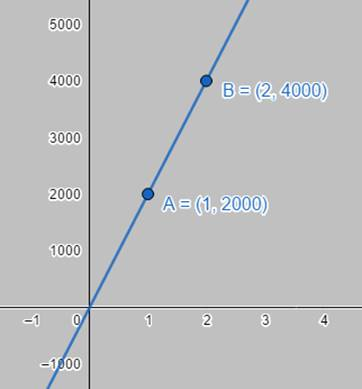PDF FILE TO YOUR EMAIL IMMEDIATELY PURCHASE NOTES & PAPER SOLUTION. @ Rs. 50/- each (GST extra)

HINDI ENTIRE PAPER SOLUTION

MARATHI PAPER SOLUTION

SSC MATHS I PAPER SOLUTION

SSC MATHS II PAPER SOLUTION

SSC SCIENCE I PAPER SOLUTION

SSC SCIENCE II PAPER SOLUTION

SSC ENGLISH PAPER SOLUTION

SSC & HSC ENGLISH WRITING SKILL

HSC ACCOUNTS NOTES

HSC OCM NOTES

HSC ECONOMICS NOTES

HSC SECRETARIAL PRACTICE NOTES

# 2019 Board Paper Solution

HSC ENGLISH SET A 2019 21st February, 2019

HSC ENGLISH SET B 2019 21st February, 2019

HSC ENGLISH SET C 2019 21st February, 2019

HSC ENGLISH SET D 2019 21st February, 2019

SECRETARIAL PRACTICE (S.P) 2019 25th February, 2019

HSC XII PHYSICS 2019 25th February, 2019

CHEMISTRY XII HSC SOLUTION 27th, February, 2019

OCM PAPER SOLUTION 2019 27th, February, 2019

HSC MATHS PAPER SOLUTION COMMERCE, 2nd March, 2019

HSC MATHS PAPER SOLUTION SCIENCE 2nd, March, 2019

SSC ENGLISH STD 10 5TH MARCH, 2019.

HSC XII ACCOUNTS 2019 6th March, 2019

HSC XII BIOLOGY 2019 6TH March, 2019

HSC XII ECONOMICS 9Th March 2019

SSC Maths I March 2019 Solution 10th Standard11th, March, 2019

SSC MATHS II MARCH 2019 SOLUTION 10TH STD.13th March, 2019

SSC SCIENCE I MARCH 2019 SOLUTION 10TH STD. 15th March, 2019.

SSC SCIENCE II MARCH 2019 SOLUTION 10TH STD. 18th March, 2019.

SSC SOCIAL SCIENCE I MARCH 2019 SOLUTION20th March, 2019

SSC SOCIAL SCIENCE II MARCH 2019 SOLUTION, 22nd March, 2019

XII CBSE - BOARD - MARCH - 2019 ENGLISH - QP + SOLUTIONS, 2nd March, 2019

# HSCMaharashtraBoardPapers2020

(Std 12th English Medium)

HSC ECONOMICS MARCH 2020

HSC OCM MARCH 2020

HSC ACCOUNTS MARCH 2020

HSC S.P. MARCH 2020

HSC ENGLISH MARCH 2020

HSC HINDI MARCH 2020

HSC MARATHI MARCH 2020

HSC MATHS MARCH 2020

# SSCMaharashtraBoardPapers2020

(Std 10th English Medium)

English MARCH 2020

HindI MARCH 2020

Hindi (Composite) MARCH 2020

Marathi MARCH 2020

Mathematics (Paper 1) MARCH 2020

Mathematics (Paper 2) MARCH 2020

Sanskrit MARCH 2020

Sanskrit (Composite) MARCH 2020

Science (Paper 1) MARCH 2020

Science (Paper 2)

Geography Model Set 1 2020-2021

MUST REMEMBER THINGS on the day of Exam

Are you prepared? for English Grammar in Board Exam.

Paper Presentation In Board Exam

How to Score Good Marks in SSC Board Exams

Tips To Score More Than 90% Marks In 12th Board Exam

How to write English exams?

How to prepare for board exam when less time is left

How to memorise what you learn for board exam

No. 1 Simple Hack, you can try out, in preparing for Board Exam

How to Study for CBSE Class 10 Board Exams Subject Wise Tips?

JEE Main 2020 Registration Process – Exam Pattern & Important Dates

NEET UG 2020 Registration Process Exam Pattern & Important Dates

How can One Prepare for two Competitive Exams at the same time?

8 Proven Tips to Handle Anxiety before Exams!